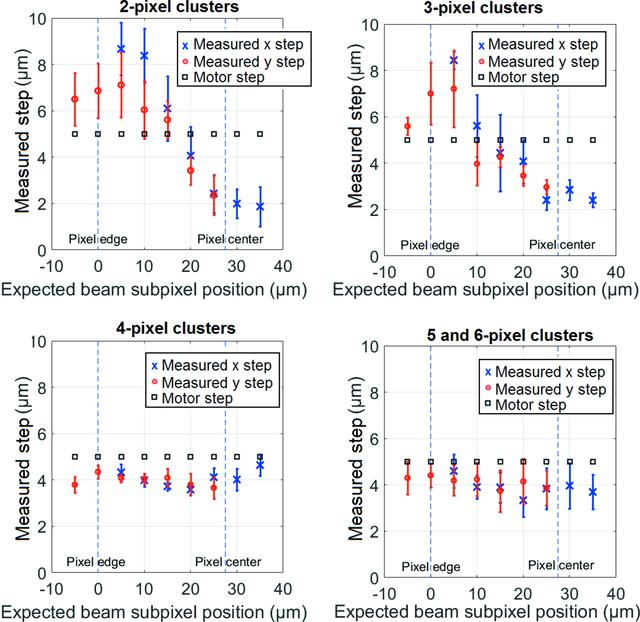disable zoom     view article Figure 7 Dependence of the measured step on the expected beam position using different pixel clusters at 120 keV. The measured steps can be inferred from Fig. 1, where the first measured step along the x direction and its error bar are the mean and standard deviation of the following steps: (1 to 2), (9 to 10), (17 to 18), (25 to 26), (33 to 34), (41 to 42), (49 to 50) and (57 to 58). Similarly, the second measured step and its error bar are the mean and standard deviation of the following steps: (2 to 3), (10 to 11), (18 to 19), (26 to 27), (34 to 35), (42 to 43), (50 to 51) and (58 to 59). The x-axis represents the expected position of the beam inside the pixel where position 0 µm is the pixel edge (pixel start) and 27.5 µm is the pixel center.JOURNAL OFSYNCHROTRONRADIATION
ISSN: 1600-5775
Volume 25| Part 6| November 2018| Pages 1650-1657